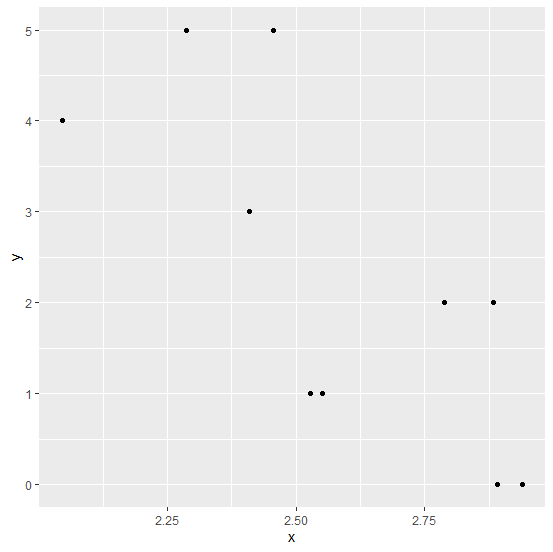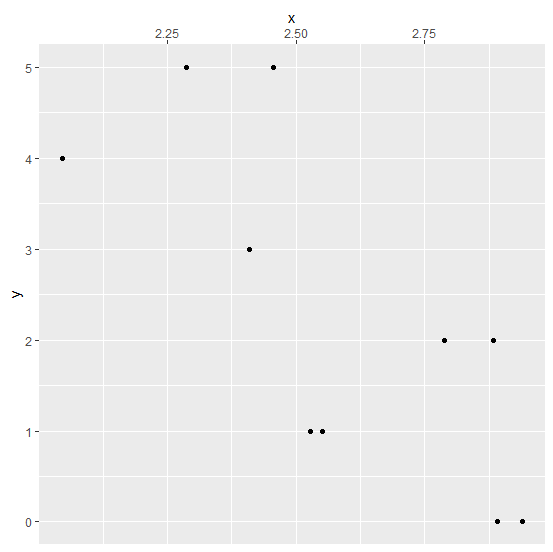# How to X-axis labels to the top of the plot using ggplot2 in R?

Usually, a plot created in R or any of the statistical analysis software have X-axis labels on the bottom side but we might be interested in showing them at the top of the plot. It can be done for any type of two-dimensional plot whether it is a scatterplot, bar plot, etc. This is possible by using scale_x_continuous function of ggplot2 package in R.

## Example

Live Demo

set.seed(123)
x<-runif(10,2,3)
y<-rpois(10,2)
df<-data.frame(x,y)
df

## Output

   x    y
1 2.287578 5
2 2.788305 2
3 2.408977 3
4 2.883017 2
5 2.940467 0
6 2.045556 4
7 2.528105 1
8 2.892419 0
9 2.551435 1
10 2.456615 5
library(ggplot2)

## Example

Creating a simple scatterplot −

ggplot(df,aes(x,y))+geom_point()

## OutputCreating a scatterplot with X-axis labels on the top of the plot −

## Example

ggplot(df,aes(x,y))+geom_point()+scale_x_continuous(position="top")

## Output